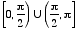# arcsec

Inverse Secant
sec-1
Sec-1
arcsec
Arcsec

The inverse function of secant.

Basic idea: To find sec-1 2, we ask "what angle has secant equal to 2?" The answer is 60°. As a result we say that sec-1 2 = 60°. In radians this is sec-1 2 = π/3.

More: There are actually many angles that have secant equal to 2. We are really asking "what is the simplest, most basic angle that has secant equal to 2?" As before, the answer is 60°. Thus sec-1 2 = 60° or sec-1 2 = π/3.

Details: What is sec-1 (–2)? Do we choose 120°, –120°, 240° , or some other angle? The answer is 120°. With inverse secant, we select the angle on the top half of the unit circle. Thus sec-1 (–2) = 120° or sec-1 (–2) = 2π/3.

In other words, the range of sec-1 is restricted to [0, 90°) U (90°, 180°] or. Note: sec 90° is undefined, so 90° is not in the range of sec-1.

Note: arcsec refers to "arc secant", or the radian measure of the arc on a circle corresponding to a given value of secant.

Technical note: Since none of the six trig functionssine, cosine, tangent, cosecant, secant, and cotangent are one-to-one, their inverses are not functions. Each trig function can have its domain restricted, however, in order to make its inverse a function. Some mathematicians write these restricted trig functions and their inverses with an initial capital letter (e.g. Sec or Sec-1). However, most mathematicians do not follow this practice. This website does not distinguish between capitalized and uncapitalized trig functions.